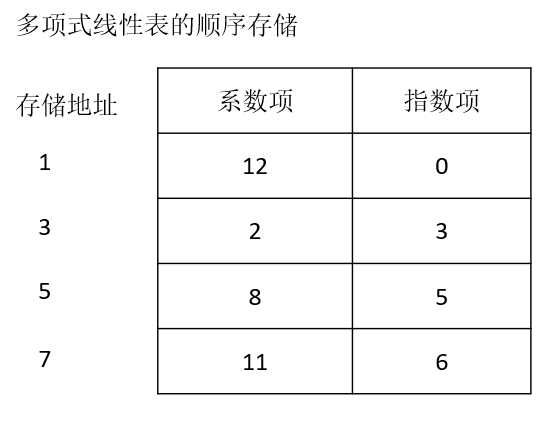Pn（x）= p0 + p1x + p2x2 + …… + pnxn

P = （p0，p1，p2，……，pn）

Q = （q0，q1，q2，q2，……，qm）

R =（p0+ q0，p1+ q1，p2+ q2，……，pm+ qm，pm+1，……，pn）

T（x）= 12x2 + 2x12000+3x30000

Pn（x）= p1xe1+ p2xe2 + …… + pmxem

0<=e1< e2<……<em=n

P = （（p1，e1），（p1，e2），……，（pm，em））

P（x）= 12 + 2x3+8x5+11x6

P = （（12，0）,（2，3），（8，5），（11，6））```package com.milihua.algorithm.lineartable;
public class PolyData {
/**
* 多项式系数项
*/
public int coef;
/**
* 多项式指数项
*/
public int expn;
/**
* 多项式项构造函数
*/
PolyData(int coef,int expn){
this.coef = coef;
this.expn = expn;
}
public int getCoef() {
return coef;
}
public void setCoef(int coef) {
this.coef = coef;
}
public int getExpn() {
return expn;
}
public void setExpn(int expn) {
this.expn = expn;
}
}```

```package com.milihua.algorithm.lineartable;

import java.util.Iterator;
import java.util.Scanner;
public class Polynomial {

/**
* 存储第一个多项式的链表
*/

/**
* 存储第二个多项式的链表
*/

/**
* 存储运算结果的多项式链表
*/

/**
* 添加数据到链表尾部
*
* @param inPolyData
* @return
*/

}

/**
* 添加数据到链表
*
* @param inPolyData
* @return
*/

}

/**
* 比较每项的指数大小
*
* @param aExpen
* @param bExpn
* @return 0两个指数相等，1第一个链表的指数大，-1第二个链表的指数大
*/

public int compExpn(int aExpen, int bExpn) {

if (aExpen == bExpn) {

return 0;

} else if (aExpen > bExpn) {

return 1;

} else {

return -1;

}

}

/**
* 两个多项式链表相加
*
* @return
*/

for (Iterator<PolyData> iter = polyListOne.iterator(); iter.hasNext();) {
PolyData poly = iter.next();
for (Iterator<PolyData> iterTwo = polyListTwo.iterator(); iterTwo.hasNext();) {
PolyData polyTwo = iterTwo.next();
switch (compExpn(poly.expn, polyTwo.expn)) {
case 0:
PolyData newPolyData = new PolyData(poly.coef + polyTwo.coef, poly.expn);
polyListTwo.remove(polyTwo);
break;
case 1:
polyListTwo.remove(polyTwo);
break;
case -1:
polyListTwo.remove(polyTwo);
break;
}
break;
}

}

}

/**
* 遍历链表并显示出来
*
* @param list
*/

for (Iterator<PolyData> iter = list.iterator(); iter.hasNext();) {
PolyData poly = iter.next();
System.out.print(poly.getCoef() + "x^" + poly.getExpn() + "+");
}
System.out.println();

}

return polyListOne;
}

this.polyListOne = polyListOne;
}

return polyListTwo;
}

this.polyListTwo = polyListTwo;
}

return polyListResult;
}

this.polyListResult = polyListResult;
}
}```

1、遍历第一个多项式；

2、在遍历过程中，处理每一个单项；

（1）遍历第二个多项式；

（2）比较两个单项式的指数；

（3）若指数相同，则两个单项式的系数相加，并形成新的单项式添加到运算结果列表中；若指数不相同，则两个单项式都添加到运算结果列表中。

PolynomialTest类为测试类，代码如下。

```package unittest;
import java.util.Scanner;
import com.milihua.algorithm.lineartable.PolyData;
import com.milihua.algorithm.lineartable.Polynomial;

public class PolynomialTest {
public static void main(String[] args) {

Polynomial  poly = new Polynomial();
//声明Scanner变量，并用new运算符实例化Scanner
Scanner sc = new Scanner(System.in);
System.out.println("----请输入第一个多项式\r\n输入方式为“系数，"
+ "指数”\r\n结束请输入0----");
while(true)
{
String  str = sc.next();
if( str.equals("0") )
{
System.out.println("----第一个多项式输入结束----");
break;
}
String[] strArray = str.split(",");
if( strArray.length < 2 )
{
System.out.println("----输入的数据格式错误----");
break;
}
int coef = Integer.parseInt(strArray);
int expn = Integer.parseInt(strArray);
PolyData polyData = new PolyData(coef,expn);

}
poly.display(poly.getPolyListOne());
System.out.println("----请输入第二个多项式\r\n输入方式为“系数，"
+ "指数”\r\n结束请输入0----");
while(true)
{
String  str = sc.next();
if( str.equals("0") )
{
System.out.println("----第二个多项式输入结束----");
break;
}
String[] strArray = str.split(",");
if( strArray.length < 2 )
{
System.out.println("----输入的数据格式错误----");
break;
}
int coef = Integer.parseInt(strArray);
int expn = Integer.parseInt(strArray);
PolyData polyData = new PolyData(coef,expn);